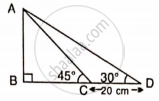# Find Ab and Bc, If: - Mathematics

Sum

Find AB and BC, if:#### Solution

Let BC = x m

BD = BC + CD = (x + 20) cm

In ΔABD,

tan 30° = "AB"/"BD"

(1)/(sqrt(3)) = "AB"/(x + 20)

x + 20 = sqrt(3)"AB"           .....(1)

In ΔABC

tan 45° = "AB"/"BC"

1 = "AB"/x

AB = x         ...(2)

From (1)

AB + 20 = sqrt(3)"AB"

AB(sqrt(3)-1) = 20

AB = (20)/((sqrt3 - 1))

= (20)/((sqrt3 - 1)) xx ((sqrt(3) + 1))/((sqrt(3) + 1))

= (20(sqrt(3)+1))/(3 - 1)

= 27.32cm

From (2)

AB = x = 27.32 cm

Therefore BC = x = AB = 27.32 cm

Therefore, AB = 27.32 cm, BC = 27.32 cm

Concept: Solution of Right Triangles
Is there an error in this question or solution?

#### APPEARS IN

Selina Concise Mathematics Class 9 ICSE
Chapter 24 Solution of Right Triangles [Simple 2-D Problems Involving One Right-angled Triangle]
Exercise 24 | Q 17.1 | Page 304